# Raffles and Strings

##### Age 16 to 18

Published 2014

Here you will find two questions, complete with hints, for you to get some practice with STEP questions. First up is a raffle problem from STEP I  2011. Then, you will be working with a question on strings from Step III in 2008.

### How many tickets will you sell? (Question 12 STEP I 2011)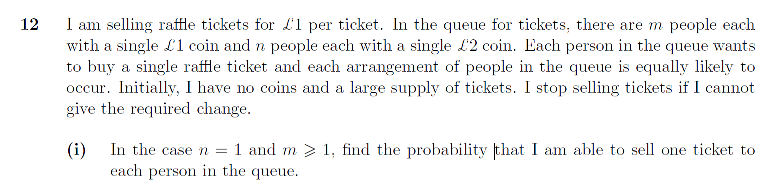When can we fail to provide change? What is the probability this happens? How is this related to the probability of successfully being able to give change to everyone?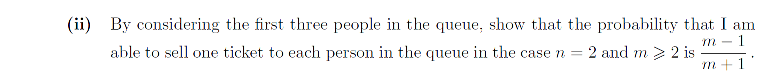What different ways can we fail now (and why do we only need to consider the first three people)? What are the probability these events occur? Again, how is this related to the chance we successfully sell tickets to everyone?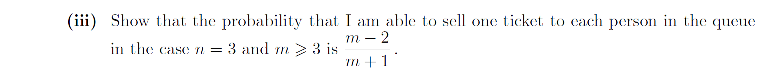How many people at the front of the queue do we need to consider now? What different scenarios mean we will fail to be able to provide change to the rest of the queue?

### How many loops will you make? (Question 13 STEP III 2008)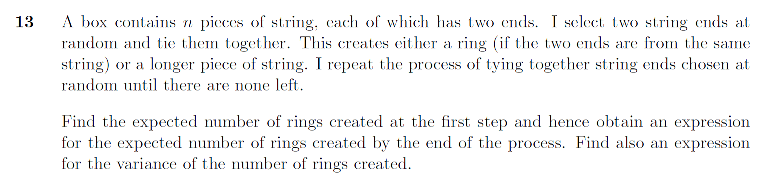What is the probability you create one ring after the first step? What about zero rings? What does this give as the expectation and variance of the number of rings?

What can you say about the number of free ends at each step? How can you use this to determine the expectation and variance?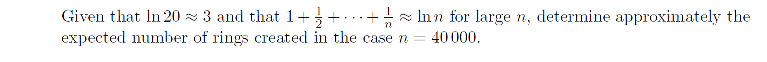What happens when you put $n=40000$ into the formula from the previous part of the question? How can you manipulate the expression to use the given approximation for $ln n$?

If after these two questions you're still itching for more practice at probability then you're in luck! Probability questions are easy to find in past papers; all you need to do is turn to Section C and you should be able to find something relevant for you to practice on.